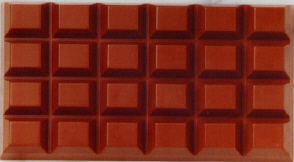© | << < ? > >> | Dror Bar-Natan: Talks:

# Kolmogorov's Solution of Hilbert's 13th Problem

### The Graduate Student Seminar Department of Mathematics, University of Toronto October 10, 2002, 12:00 Noon, SS 5017B

Are there any truly new continuous functions of two variables, beyond addition and functions of one variable? Well, multiplication isn't one, for multiplication is a simple composition of addition and of functions of just one variable: xy = exp(log x + log y). Powers and logarithms wouldn't do either, as xy = exp(exp(log y + log log x)) and logx y = exp(log log y + (- log log x)). Trig functions won't do, they are functions of one variable. Maybe Bessel functions? Anybody has a clue what they are? Anyway, according to Kolmogorov and Arnol'd, we need not worry, for the following amazing theorem holds true:

Theorem. Any real-valued continuous function on a compact set in Rn is a finite composition of (several instances of) the binary function "+" and of single-variable continuous functions.

On top of being a beauty, this theorem also resolves the 13th of Hilbert's famed 23 problems, in which he presented a certain specific function of three variables and asked if it can be re-written as a composition of continuous functions of two or less variables. Well, our theorem does a lot better.

Ok, if you can't make it to the lecture or if you want to do some work in advance, just do some exercises!A chocolate tablet from
http://www.vantagehouse.com/hans/hb_pralines.htm